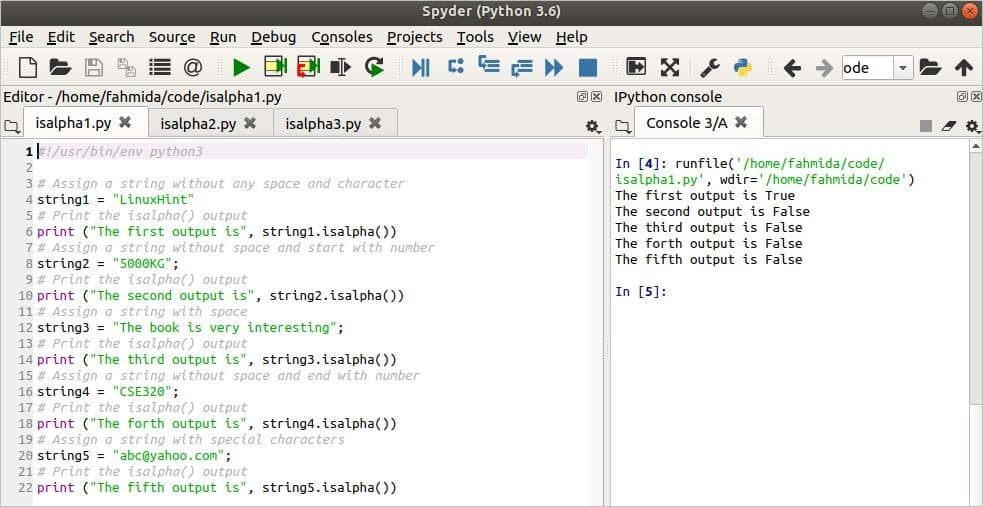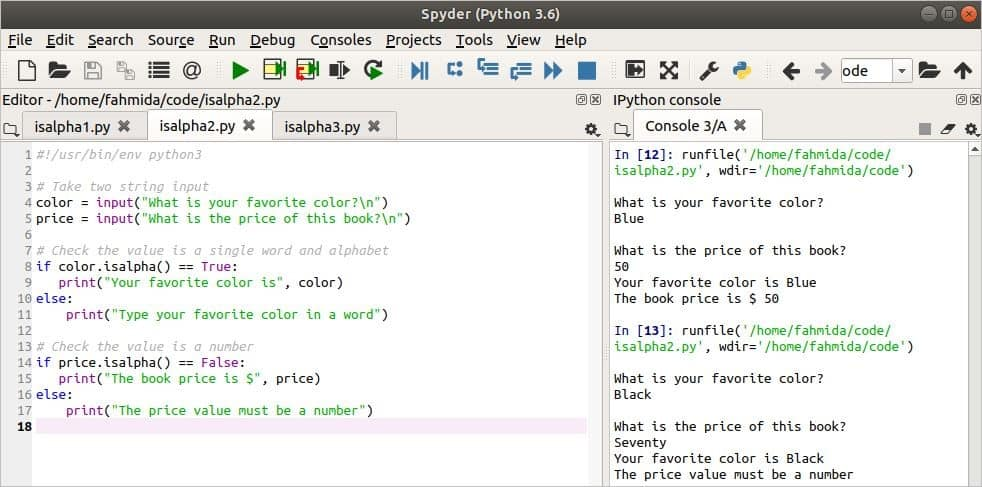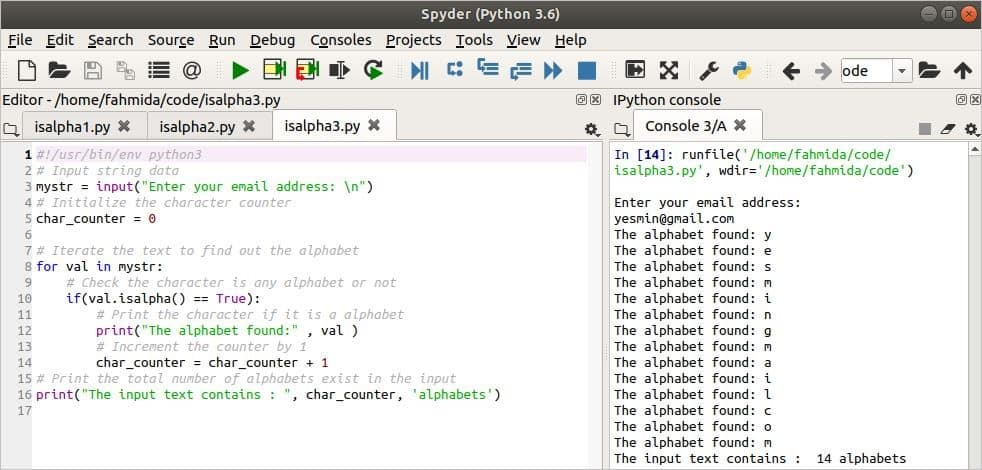Python

# How to Use the Python Isalpha Function

Sometimes, we need to check the content of data for programming purposes. There are many different types of built-in functions in Python for string data to check the content This content may include letters, numbers, or other special characters. The isalpha() function is one of the useful built-in functions of Python that can be used to find out whether or not the content of the data is alphabetic. This function searches the alphabet in the starting of the string value. If the starting value of the string is a letter, then this function returns true; otherwise, it returns false. This tutorial will show you how to can use the isalpha() function in Python.

### Syntax

string.isalpha()

Here, the string will contain any string data. The isalpha() function has no argument and will check whether or not the data in the string includes letters.

### Example 1: Simple Use of the isalpha() Function

In the following example, the isalpha() function is applied to five different types of string data. The value of the string1 variable is a text of a single word that contains all alphabetic characters. The value of the string2 variable is a text of a single word that contains numbers at the beginning of the text. The value of the string3 variable is a text of multiple words. The value of the string4 variable is a text of a single word that contains the number at the end of the text. The value of the string5 variable is a text of a single word that contains special characters and alphabetic letters.

#!/usr/bin/env python3

# Assign a string without any space and character
string1 = "LinuxHint"
# Print the isalpha() output
print ("The first output is", string1.isalpha())
string2 = "5000KG";
# Print the isalpha() output
print ("The second output is", string2.isalpha())
# Assign a string with space
string3 = "The book is very interesting";
# Print the isalpha() output
print ("The third output is", string3.isalpha())
# Assign a string without space and end with number
string4 = "CSE320";
# Print the isalpha() output
print ("The forth output is", string4.isalpha())
# Assign a string with special characters
string5 = "abc@yahoo.com";
# Print the isalpha() output
print ("The fifth output is", string5.isalpha())

Output

The following output will appear after running the script. The first output is true because all characters of the text are alphabetic letters. The second output is false because the text contains numeric characters at the beginning. The third output is false because the text contains multiple words with spaces. The fourth output is false because the text contains the number character at the end. The fifth output is false because the text contains special characters.### Example 2: Validate Data with the isalpha() Function

You can use the isalpha() function to validate any data you might need for programming purposes. This process is shown in the following script. Here, two string values will be taken from the users. The isalpha() function is used to validate that the first input value is a string of alphabets and the second input value is a number. The isalpha() function returns true for any text if the content of the text is all alphabetic characters. The isalpha() function returns false if any character of the text is not an alphabetic character.

#!/usr/bin/env python3

# Take two string input
color = input("What is your favorite color?\n")
price = input("What is the price of this book?\n")

# Check the value is a single word and alphabet
if color.isalpha() == True:
else:
print("Type your favorite color in a word")

# Check the value is a number
if price.isalpha() == False:
print("The book price is", price)
else:
print("The price value must be a number")

Output

The above script is run two times with the valid data and the invalid data. The first time, the valid data are passed for both inputs and it shows the output properly. The second time, invalid data is passed for the second input and an error message is printed as this output.### Example 3: Count the Total Number of Alphabets in a Text

The isalpha() function is used in the following script to count the total number of characters that are alphabetical in the given text. An email address will be taken as input and stored in the variable, mystr. In this example, the char_counter variable is used to count the total number of alphabetic characters in the mystr. This variable is initialized to 0 and each time that an alphabetic character is found in the mystr, the char_counter will be incremented by one. The for loop is used here to read each character of the mystr, while the isalpha() function is used to check whether or not the character is alphabetic.

#!/usr/bin/env python3
# Input string data
# Initialize the character counter
char_counter = 0

# Iterate the text to find out the alphabet
for val in mystr:
# Check the character is any alphabet or not
if(val.isalpha() == True):
# Print the character if it is a alphabet
print("The alphabet found:" , val )
# Increment the counter by 1
char_counter = char_counter + 1
# Print the total number of alphabets exist in the input
print("The input text contains : ", char_counter, 'alphabets')

Output

The output shows that yesmin@gmail.com is taken as the input value after running the script.  The input value contains two special characters (‘@’ and ‘.’), and the remainder of the characters are alphabetic.  So, the input text contains 14 alphabetic letters, after omitting the special characters.### Conclusion

It is essential to check the content of any text or variable before solving many programming problems. Python contains several built-in functions, such as isnumeric() , isdigit(), isalnum(), isdecimal(), isalpha(), and others, to check the content of the string data. The different uses of the isalpha() function are explained in this tutorial by using simple examples. This should help new Python users to understand the purposes of using the isalpha() function and others like it.